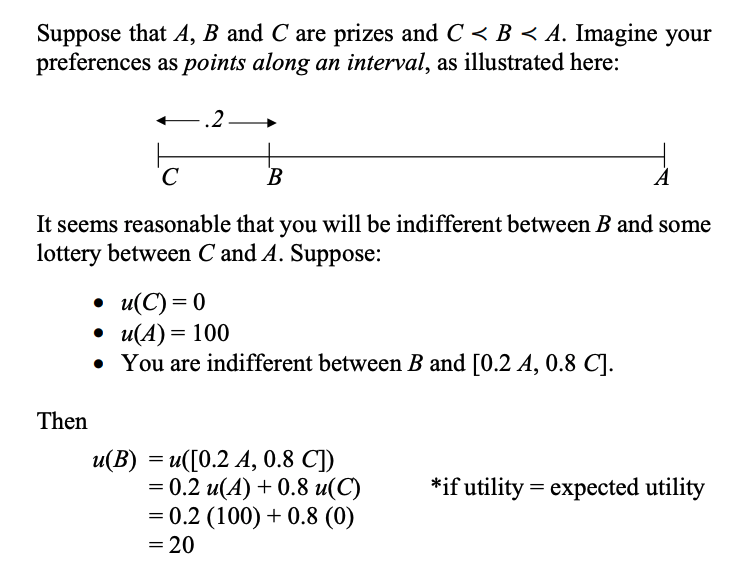Any interval scale can be linearly transformed (ordinal transformation) between each other and not be affected

Assign to each outcome a value such that and

## von Neumann-Morgenstern Theorem (vNM) §

We need interval scales for most of the rules for DUI, and we need them for DUR. vNM tells us how to construct an interval utility scale.

We find your utility for by measuring the risks that you are willing to take to get . vNM shows that your utility for a lottery is equal to its expected utility.

If your preferences have enough structure (i.e., if they satisfy the vNM conditions), then they can be represented by a utility function u (unique up to positive linear transformation) which has the expected utility property.

### Lotteries §

• We have a set of basic prizes/outcomes
• We can compose lotteries (if and are lotteries, then so is )
• This creates compound lotteriesPeterson argues that vNM is ok for descriptive decision theory but not normative decision theory (vNM does not distinguish the meaning of utility from the measurement of utility)

Normative decision theory involves prescribing actions which needs a strong version of EU max: acts are rational because they maximize expected utility. This demands a concept of utility that is independent from the measurement of utility itself (or we get a circular argument)

### Axioms §

1. vNM 1, Completeness: or or
2. vNM 2, Transitivity: if and then
3. vNM 3, Independence: if and only if
4. vNM 4, Continuity: if there such that
5. vNM 5, Probability: it does not matter if you are awarded prize A if you first roll a die and then roll it again, or make a double roll, provided that you only get the prize if you get two sixes
1. If then